## Section A midterm 2

1. A square loop of wire with sides of length L = 0.17 m and total resistance R = 50 ohms is moving with constant velocity v into a region of constant magnetic field B = 0.76 T. The magnetic field is perpendicular to the plane of the loop and into the page as shown in the sketch. An induced current of I = 0.043 amperes is flowing around the loop.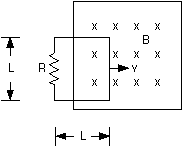[5 points] (a) Which way will the induced current flow in the loop?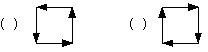The loop is moving into the field, which points into the page. Lenz's law says that if the magnetic flux through the loop is increasing into the page, the loop will set up a flux out of the page to try to cancel the change. Current flowing counter-clockwise around the loop generates a magentic field out of the page (the right-hand rule can be used to determine this - if you curl your fingers on your right hand in the direction of the current, your thumb points in the direction of the field produced by that current).

The first picture, with current counter-clockwise, is correct.

[12 points] (b) What is the induced emf that is generated in the loop and causes the current I to flow?

Although this is an induced emf problem, there isn't enough information here to solve for the induced emf using an induced emf equation. Fortunately, it's easy because we know both the current and resistance in the loop, so we can apply the familiar equation from circuits:

emf = I R = 0.043 (50) = 2.15 volts.

[8 points] (c) What is the value of the constant velocity v of the loop?

Now we can apply an induced emf equation. The easiest one to use here is the motional emf equation:

emf = vBL

v = emf / (BL) = 2.15 / [(0.76) (0.17)] = 16.6 m/s.

2. This problem deals with light passing through a succession of polarizers as indicated in the sketch: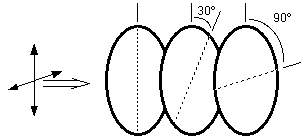[5 points] (a) Unpolarized light, with an intensity of 1000 W/m2 is incident on the first polarizer. What is the intensity of the light transmitted by the first polarizer?

When unpolarized light passes through a polarizer, the intensity is cut in half. Therefore, the transmitted intensity is 500 W / m2.

[7 points] (b) The light now passes through a second polarizer, which has its transmission axis rotated by 30 degrees relative to the axis of the first polarizer. What is the intensity of the light transmitted by the second polarizer?

Applying Malus' law, the intensity after going through the second polarizer is:

S2 = S1 cos230 = 500 (0.75) = 375 W / m2.

[8 points] (c) The light transmitted by the second polarizer now encounters a third polarizer, which has its transmission axis oriented at 90 degrees relative to the first polarizer. What is the intensity of the light transmitted by the third polarizer?

Again, apply Malus' law. The angle that goes in is the difference between the angles for the second and third polarizers, 60 degrees. This is because the second polarizer produces light polarized at 30 degrees to the vertical. It's the angle between that polarization direction and the polarization axis of the third polarizer that's needed.

S3 = S2 cos260 = 375 (0.25) = 93.8 W / m2.

[5 points] (d) Suppose the second polarizer is removed. What is the intensity of light transmitted by the two (i.e., first and third) polarizers now?

Zero.Light passed by the first polarizer is polarized along the vertical direction. Without the second polarizer to rotate the polarization direction, none of the vertically-polarized light gets through the third polarizer, which passes light polarized in the horizontal direction.

3.

[5 points] (a) An object is placed 20 cm from a concave mirror, forming a real image. The image position is marked, and the object is now moved and placed there. Where will the image be now? Briefly explain your reasoning.

The image will be where the object was originally, 20 cm from the mirror. If you draw a ray diagram for the first case, the diagram in the second case will be identical, with the directions of the rays all reversed. When real images are involved, the object and image are interchangable.

[8 points] (b) An object is placed 12 cm from a convex mirror with a focal length of -15 cm. On the diagram, sketch the ray diagram showing the position of the image.The parallel ray goes from the tip of the object, parallel to the principal axis, and reflects off the mirror so that when you extend the reflected ray back it passes through the focal point.
The chief ray heads toward C, and comes straight back.
The focal ray heads toward F, and reflects off the mirror parallel to the principal axis.

Extending all three reflected rays back gets you the point where they cross; that's where the tip of the image is.

[6 points] (c) Where is the image located?

Re-arranging the mirror equation to solve for the image distance gives:

di = do f / (do - f) = (12) (-15) / (12 - -15)

This gives an image distance of -6.67 cm.

[4 points] (d) What are the image characteristics? (Is the image real or virtual? Upright or inverted?)

The image is virtual and upright.

[2 points] (e) If the image is 4 cm tall, how tall is the object?

hi = ho (-di / do) = 4 (6.67 / 12) = 2.22 cm.

4. A light wave in air has a frequency of 6.0 x 1014 Hz.

[3 points] (a) What is its wavelength?

With an index of refraction of 1.0, the velocity of light in air is v = c = 3.0 x 108 m/s. Therefore:

wavelength = v / f = 3.0 x 108 / 6.0 x 1014 = 5.0 x 10-7 m.

[3 points] (b) The light wave now enters a block of glass, with refractive index n = 1.5. What is the frequency of the light wave now?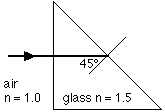The frequency is the one thing that stays the same, so it's still 6.0 x 1014 Hz.

[3 points] (c) What is the wavelength of the light wave in glass?

You can figure out the new speed and then apply the equation from part (a), or use the equation: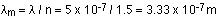The wavelength without the subscript is the wavelength in vacuum (air is being treated the same as vacuum for the purposes of this question); the wavelength with the subscript m is the wavelength in the medium.

[7 points](d) The light ray is now incident on a glass-air interface, with an angle of incidence in the glass of 45 degrees. Does the light ray emerge from the glass into air? Explain your answer.

The ray is trying to pass from a higher-n medium to a lower-n medium. Whenever this happens, calculate the critical angle to see if total internal reflection occurs: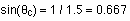This gives a critical angle of 41.8 degrees. The angle of incidence (45°) is larger than the critical angle, so the light is totally reflected internally. No light emerges from the glass into the air here.

[9 points](e) The far side of the glass block is immersed in water, with a refractive index of 1.33. The light ray is again incident on the interface between glass and water, with an angle of incidence in the glass of 45 degrees. Does the light ray now emerge from the glass block into the water? If so, what is the angle of the refracted ray from the normal to the water-glass interface?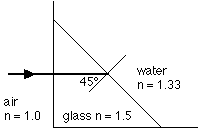Again, work out the critical angle:The critical angle is now 62.5 degrees. The angle of incidence is less than this, so there will be a refracted ray. Snell's law can be used to get the angle of refraction:Taking the inverse sine gives an angle of refraction of 52.9°.